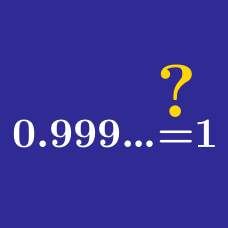Algebra

# Algebra Missteps

What are the solution(s) to the equation $\sqrt{x+ 4} = x -2?$

(Partial solution)
The equation can be rewritten as follows: \begin{aligned} \sqrt{x+ 4} &= x -2\\ x+ 4 &= (x -2)^2\\ x+ 4 &= x^2 - 4x + 4\\ 0 &= x^2 - 5x\\ 0 &= x(x - 5). \end{aligned}

Where is the error in the solution to this problem?

Find the solution set of $20 - x > 5$.

Line 1. $\,\,\,\,\,20 - x > 5$

Line 2. $\,\,\,\,\,-x > -15$

Line 3. $\,\,\,\,\,x >15$

Where is the error in the solution to this problem?

Find the solution set of $3 - x < 8$.

Step 1. $\,\,\,\,\,3 - x < 8$

Step 2. $\,\,\,\,\,-x < 5$

Step 3. $\,\,\,\,\,x < -5$

Is the following solution valid?

1. $\,\,\,\,\,\sqrt{x - 5} = -3$

2. $\,\,\,\,\,x - 5 = 9$

3. $\,\,\,\,\,x = 14$

Consider the following work done to solve the equation $\sqrt{x + 1} = 1.$

1. $\,\,\,\,\,\sqrt{x} + \sqrt{1} = 1$

2. $\,\,\,\,\,\sqrt{x} + 1 = 1$

3. $\,\,\,\,\,\sqrt{x} = 0$

4. $\,\,\,\,\,x = 0$

×